American Journal of Theoretical and Applied Statistics
Volume 4, Issue 5, September 2015, Pages: 317-321

Study of Multivariate Data Clustering Based on K-Means and Independent Component Analysis

Md. Shamim Reza, Sabba Ruhi

Department of Mathematics, Pabna University of Science & Technology, Pabna, Bangladesh(Md. S. Reza)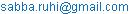(S. Ruhi)

Md. Shamim Reza, Sabba Ruhi. Study of Multivariate Data Clustering Based on K-Means and Independent Component Analysis. American Journal of Theoretical and Applied Statistics. Vol. 4, No. 5, 2015, pp. 317-321. doi: 10.11648/j.ajtas.20150405.11

Abstract: For last two decades, clustering is well-recognized area in the research field of data mining. Data clustering plays the major research at pattern recognition, Signal processing, bioinformatics and Artificial Intelligence. Clustering process is an unsupervised learning techniques where it generates a group of object based on their similarity in such a way that the objects belonging to other groups are similar and those belonging to other are dissimilar. This paper analysis the three different data types clustering techniques like K-Means, Principal components analysis (PCA) and Independent component analysis (ICA) in real and simulated data. The recent developments by considering a rather unexpected application of the theory of Independent component analysis (ICA) found in data clustering, outlier detection and multivariate data visualization. Accurate identification of data clustering plays an important role in statistical analysis. In this paper we explore the connection among these three techniques to identify multivariate data clustering and develop a new method k-means on PCA or ICA then the result shows that ICA based clustering performs well than others.

Keywords: Clustering, K-means, PCA, ICA

1. Introduction

Data clustering is an active research area for exploring and grouping set of data objects into multiple groups or clusters so that objects within the cluster have high similarity, but are very dissimilar to objects in the other clusters. It is probably fair to say that in the last 10 years, clustering techniques has become a standard tool in data mining and in the field of artificial intelligence . There are various clustering algorithms have been developed and are categorized from several aspects such as partitioning methods, hierarchical methods, density-based methods, and grid-based methods [1,3,7]. In partitioning method, a division data objects into non-overlapping clusters such that each data object is in exactly one subset. Most common partitioning method is K-means and mixture models . The recent developments by considering a rather unexpected application of the theory of Independent component analysis (ICA) found in multivariate data analysis such as outlier detection, data clustering, data visualization etc [25,27].

Independent component analysis (ICA) is a Statistical and computational technique in which the goal is to find a linear projection of the data that the source signals or components are statistically independent or as independent as possible. Among its numerous applications, ICA is the most natural tool for BSS  in instantaneous linear mixtures when the source signals are assumed to be independent. The plausibility of the statistical independence assumption in a wide variety of fields, including telecommunications, finance and biomedical engineering, helps explain the arousing interest in this research area witnessed over the last two decades. Many methods for data clustering try to identify cluster. Data clustering is carried out through the use of Principal Components Analysis (PCA) . PCA is a dimension reduction procedure where some of the variables are highly correlated with each other. If this is to be used in a contaminated data, the nature of the estimated principal components may behave differently, implemented the principal components as a multivariate data clustering method.

Data analysis methods are essential for analyzing the ever-growing enormous quantity of high dimensional data. Some literatures of cluster analysis [4,8,10] attempts to pass through data quickly to gain first order knowledge by partitioning data points into disjoint groups such that data points belonging to same cluster are similar while data points belonging to different clusters are dissimilar. One of the most popular and efficient clustering methods is the K-means method [7,18,19] which uses prototypes to represent clusters by optimizing the squared error function.

On the other end, high dimensional data are often transformed into lower dimensional data via the principal component analysis (PCA)  where coherent patterns can be detected more clearly. Such unsupervised dimension reduction is used in very broad areas such as neural networks, image processing, genomic analysis, and information retrieval. It is also common that PCA is used to project data to a lower dimensional subspace and K-means is then applied in the subspace . In other cases, data are embedded in a low-dimensional space such as the Eigen space of the graph K-means is then applied . The main basis of PCA-based dimension reduction is that PCA picks up the dimensions with the largest variances. Mathematically, this is equivalent to finding the best low rank approximation of the data via the singular value decomposition . However, this noise reduction property alone is inadequate to explain the effectiveness of PCA.

In this article, we will begin a general description of clustering, briefly describing the most popular methods of multivariate data clustering such as \k-means, PCA, ICA and proposed K-means on PCA and K-means on ICA. Finally, we compare their performance among other clustering techniques that found best in the literature.

2. Data Clustering Techniques

2.1. K-means Clustering

K-Means is one of simplest method among all other partitioning based data clustering methods [1,3,7]. Every cluster is assigned with centroids. It is a partition method technique which finds mutual exclusive clusters of spherical shape. It generates a specific number of disjoint, flat (non-hierarchical) clusters. Statically method can be used to cluster to assign rank values to the cluster categorical data. Here categorical data have been converted into numeric by assigning rank value . K-Means algorithm organizes objects into k-partitions where each partition represents a cluster. We start out with initial set of means and classify cases based on their distances to their centers. Next, we compute the cluster means again, using the cases that are assigned to the clusters; then, we reclassify all cases based on the new set of means. We keep repeating this step until cluster means don’t change between successive steps. Finally, we calculate the means of cluster once again and assign the cases to their permanent clusters.

Algorithm k-means

a)  Decide on a value for K, the number of clusters.

b)  Initialize the K cluster centers.

c)  Decide the class memberships of the N objects by assigning them to the nearest cluster center.

d)  Re-estimate the K cluster centers, by assuming the memberships found above are correct.

e)  Repeat 3 and 4 until none of the N objects changed membership in the last iteration.

The strength of K-Means clustering is relatively efficient scalable process for huge sum of data sets and easy to understand and implement. It has some drawbacks that process begins only after the mean of a cluster is initialized, user defined clusters constant and hard to handle data with noise and outliers .

2.2. Principal Component Analysis

Principal component analysis or PCA is one of the key tools in multivariate statistical analysis and is often used to reduce the dimension of data for easy exploration. As a multivariate analysis technique for dimension reduction, it aims to compress the data without losing much information the original data contains. The process of how PCA is done here is based on Johnson, R. [2,3]. It is concerned with explaining the variance-covariance structure of a set of variables through a few new variables. All principal components are particular linear combinations of the p random variables with three important properties which are:

1.  The principal components are uncorrelated.

2.  The first principal component has the highest variance; the second principal component has the second highest variance, and so on.

3.  The total variation in all the principal components combined is equal to the total variation in the original variables.

Mathematically,

Let X and Y are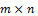matrices related by a linear transformation P. X is the original recorded data set and Y is a representation of that data set.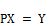(1)

Equation 1 represents a change of basis and thus can have many interpretations.

1.  P is a matrix that transforms X into Y.

2.  Geometrically, P is a rotation and a stretch which again transforms X into Y.

3.  The rows of P,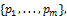are a set of new basis vectors for expressing the columns of X. Where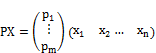We can note the form of each column of Y. The new variable Y is linear combination of original variables X.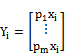The first PC is the linear combination of the variables that explains the greatest amount of the total variation in x. The second PC is the linear combination of the variables that explains the next largest amount of variation and is uncorrelated with the first PC, and so on. If the first few (say, three) components contain most of the total variation (say, 85%), then the original variables can be replaced by these components without too much loss of variance information. The principal components are computed from an eigen analysis of the covariance matrix or the correlation matrix, but results from the covariance matrix and the correlation matrix are usually not the same. If the variables are measured on scales with widely different ranges or if the units of measurement are not commensurate, it is better to perform PCA on the correlation matrix. The observations that are cluster with respect to the first few principal components or the major principal components usually correspond to cluster on one or more of the original variables. It is well known that PC’s are uncorrelated but doesn’t grantee the independence among PC’s. In such situation a recently developed techniques ICA can be a more powerful tool than PCA, where IC’s are independent.

2.3. Independent Component Analysis

Independent component analysis (ICA) is a statistical method used to discover hidden factors (sources or features) from a set of measurements or observed data such that the sources are maximally independent. The ICA algorithms are able to separate the sources according to the distribution of the data. Independent component analysis (ICA) , and projection pursuit (PP) , are closely related techniques, which try to look for interesting directions (projections) in the data. To achieve separation of mixed data into independent components ICA exploits the independence between the sources in order to achieve their separation from mixed data. In order to formally define ICA model, consider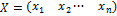as a random vector, representing n sensor signals that are observable, and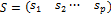as a random vector of latent mutually independent sources, where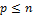.The ICA model is then given by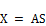Where A is a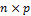matrix with full column rank, called the mixing matrix. A is assumed to be fixed, but unknown. ICA consists of estimating both the matrices A and S, when only X is known, i.e., finding a matrix W such that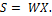Here, S is obtained by ICA based on the following two main assumptions on each source signals Si in S: i) Si is statistically independent of Sj in S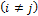, ii) Si is non-Gaussian random variable.

Although numerous application of ICA in different fields but its main drawback to determine order of IC’s . In principal component analysis, PC’s are ordered by Eigen value where first Eigen value is first pc, second Eigen value second pc and so on. But in independent component analysis, these components have no order [9,25].For practical reasons to define a criterion for sorting these components to our interest. One measurement which can match our interest very well, is kurtosis. Kurtosis is a classical measure of non-Gaussianity, and is computationally and theoretically relatively simple. From purely Gaussian distributed data, no unique independent components can be extracted; therefore, ICA should only be applied to data sets where we can find components that have a non-Gaussian distribution. Examples of super-Gaussian distributions (highly positive kurtosis) are speech signals, because these are predominantly close to zero. However, for outlier identification super Gaussian distributions (positive kurtosis) are more interesting. Negative kurtosis can indicate a cluster structure or at least a uniformly distributed factor [21,27]. Thus the components with the most negative kurtosis can give us the most relevant information. Since most negative kurtosis indicates cluster structure and highest positive kurtosis identify multivariate outlier .

3. Proposed Method

To overcome the limitations of K-Means clustering and PCA based clustering. We take an attempt to identify multivariate data clustering new approach K-means on PCA and ICA on PCA method. The main basis of PCA-based dimension reduction is that PCA picks up the dimensions with the largest variances that are PCA is used to project data to a lower dimensional subspace and K-Means; ICA is then applied in the subspace. Improving the K-Means clustering and PCA based clustering using ICA are given following steps

Step-1: By applying PCA of original data and we have to assume that the most interesting information is directly related to the highest variance in the data. After applying PCA we have to retain about 80% variability of the total variation.

Step-2: We then apply K-Means clustering method on PCA and second method can consider ICA on PCA.

Step-3: Minimize the dependence using ICA then define a criterion for sorting these components to our interest using Kurtosis. Since negative kurtosis can indicate a cluster structure or at least a uniformly distributed factor. Thus in this stage the components should consider with the most negative kurtosis to identify cluster.

4. Application

4.1. Real Data (Iris & Crabs Data, Data Source: R)

This famous (Fisher's or Anderson's) iris data set gives the measurements in centimeters of the variables sepal length and width and petal length and width, respectively, for 50 flowers from each of 3 species of iris. The species are Iris setosa, versicolor, and virginica. The Iris dataset is a well-known dataset for classification of various data clustering techniques . In Iris data first three PC’s can explain 99% variability of the total variation. So we plot first PC’s that chosen according to Eigen value and comparing their performance IC’s. Since IC’s has no order. For this reason we used kurtosis measure to ordering independent components where highest negative kurtosis considered IC1, second largest negative kurtosis consider IC2 and so on.

It is well known that iris data has three cluster based on species . From Fig.-1 and Fig.2, by applying K-Means and PCA to the total data, the graph shows two pc’s and K-Means wrongly identified three cluster but in IC’s, and ICA on PC’s gives strongly identified three cluster of Iris data.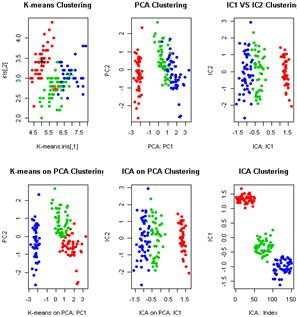Fig. 1. on the left, by applying K-Means and PCA to the total data, the result is worse than the result of ICA. However, by using PCA for preprocessing before applying K-Means and ICA, a more strongly cluster can be identified. It is well known that iris data has three cluster based on species. On the left, by applying K-Means and PCA to the total data, the graph shows two pc’s wrongly identified three cluster but in IC’s, and ICA on PC’s gives strongly identified three cluster.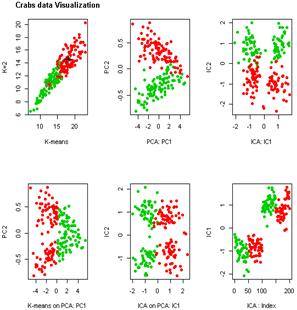Fig. 2. On the left, by applying K-Means and PCA to the crab’s data, the result is worse than the result of ICA. However, by using PCA for preprocessing before applying K-Means and ICA, a more strongly cluster can be identified.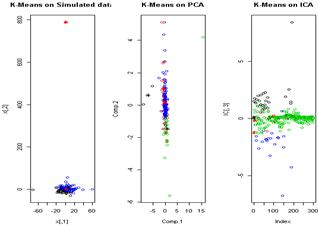Fig. 3. On the left, by applying K-Means and PCA to the simulated data and plotting first two PC’s found that PC’s couldn’t identify cluster properly where in K-means on PCA and K-Means on ICA detect three cluster from simulated data.

4.2. Simulation Study

In this section we conducted a simulation study and generate 4 variables each has 300 observations. We randomly generate a multivariate data where first variable come from normal distribution with mean 0 and standard deviation (S.D) 1, second variable from log-normal with mean 1, S.D 0.3, third from student-t distribution with parameter 1, and fourth variable from Chi-square distribution with parameter 2. According to our method, at first we apply K-Means and PCA to the simulated data where 4 variables each 300 observations. By applying K-Means clustering we define K=4 and found four cluster each size 2, 2, 171 and 125 respectively. By applying PCA and we found that first 3 PC’s can explain 84% variability of the total variation, and then we apply K-Means to the PCA scores then 4 cluster generated each of size 174, 1, 123, 2 and K-Means applying to ICA scores produced three cluster in the fig-3. The kurtosis of IC’s is 18.22, 122.67, 26.30 and 282.40 respectively. From kurtosis value of components lowest kurtosis value 18.22 treated as IC1 and second lowest kurtosis value 26.30 treated as IC2 and so on. Since most negative kurtosis indicates cluster structure and highest positive kurtosis identify multivariate outlier.

5. Conclusion

In this paper we have considered three techniques to identify multivariate data cluster includes K-Means, PCA and ICA. We also applied a new and novel method K-Means on PCA and K-Means on ICA for multivariate data clustering. To overcome ordering independent components we used classical measure of kurtosis, we then apply our measure to Iris data, Crabs data and simulated data, and try to examine the capacity of K-Means, PCA, and K-Means on PCA, ICA and ICA on PCA for finding cluster through normal dot plot. In both data sets, our proposed method K-Means on PCA and ICA a new visualization technique correctly diagnosis clusters than only PCA or only K-Means. Although in our study, we considered partitioned methods to identify cluster. In future we have to use hierarchical methods, density-based methods, and grid-based methods and comparing their performance ICA based techniques and tries to define appropriate classifier with confidence interval.

Acknowledgment

My sincere appreciation and thanks also go to the colleagues of Mathematics Department at Pabna University of Science & Technology for their unreserved knowledge sharing and cooperation.

References

1. Bradley, P., & Fayyad, U. (1998). Refining initial points for k means clustering. Proc. 15th International Conf. on Machine Learning.
2. Cluster R package.(http://cran.r-project.org/web/packages/ cluster/index.html).
3. Ding, C., & He, X.. K-Means clustering via principal component analysis. Computational Research Division, Lawrence Berkeley National Laboratory, Berkeley, CA 94720
4. Duda, R. O., Hart, P. E., & Stork, D. G. (2000). Pattern classification, 2nd ed. Wiley.
5. Eckart, C., & Young, G. (1936). The approximation of one matrix by another of lower rank. Psychometrika, 1,183–187.
6. Groeneveld RA (1998) A class of quantile measures for kurtosis. Am Stat 52: 325-329.
7. Hartigan, J., & Wang, M. (1979). A K-means clustering algorithm. Applied Statistics, 28, 100–108.
8. Hastie, T., Tibshirani, R., & Friedman, J. (2001). Elements of statistical learning. Springer Verlag.
9. Hyv¨arinen, A. and Oja, E.: Independent component analysis: Algorithms and applications. Neural Networks. 4-5(13):411-430. 2000.
10. Jain, A., & Dubes, R. (1988). Algorithms for clustering data. Prentice Hall.
11. J.C. Salagubang and Erniel B. Barrios, Outlier detection in high dimensional data in the context of clustering,12th National Convention on Statistics (NCS) EDSA Shangri-La Hotel, Mandaluyong City October 1-2, 2013
12. Johnson, R. and Wischern, D. (2002). Applied Multivariate statistical analysis, 5th ed. Prentice-Hall, Inc.
13. Jolliffe, I. (2002). Principal component analysis. Springer. 2nd edition.
14. Jones,M. and Sibson, R. What is projection pursuit? J. of the Royal Statistical Society, Ser. A, 150:1-36. 1987.
15. Kotz, S., and Seier, E. (2008), Kurtosis of the Two-Sided Power Distribution, Brazilian Journal of Probability and Statistics, 28, 6168.
16. Leela, V. K. Sakthi priya and R. Manikandan, 2013. "Comparative Study of Clustering Techniques in Iris Data Sets"World Applied Sciences Journal 29 (Data Mining and Soft Computing Techniques): 24-29, 2014 ISSN 1818-4952.
17. Lihua An, S.Ejaz Ahmed. Improving the performance of kurtosis estimator. Computational Statistics and Data Analysis 52, 2669-2681. 2008.
18. Lloyd, S. (1957). Least squares quantization in pcm. Bell Telephone Laboratories Paper, Marray Hill.
19. MacQueen, J. (1967). Some methods for classification and analysis of multivariate observations. Proc. 5th Berkeley Symposium, 281–297.
20. Maurya V.N., Misra R.B., Jaggi C.K., and Maurya A.K., Performance analysis of powers of skewness and kurtosis based multivariate normality tests and use of extended Monte Carlo simulation for proposed novelty algorithm, American Journal of Theoretical and Applied Statistics, Science Publishing Group, USA, Vol. 4(2-1), pp. 11-18, 2015.
21. Matthias Scholz, Yves Gibon, Mark Stitt and Joachim Selbig, Independent component analysis of starch deficient pgm mutants. Proceedings of the German conference on Bioinformatics. Gesellschaft fur info mark, Bonn, pp.95-104,2004.
22. Meira Jr., W.; Zaki, M. Fundamentals of Data Mining Algorithms.(http://www.dcc.ufmg.br/miningalgorithms/DokuWiki/doku.php).
23. Ng, A., Jordan, M., & Weiss, Y. (2001). On spectral clustering: Analysis and an algorithm. Proc. Neural Info. Processing Systems (NIPS 2001).
24. Pearson K (1905) Skew variation, a rejoinder. Biometrika 4:169212.
25. Reza, M.S., Nasser, M. and Shahjaman, M. (2011) An Improved Version of Kurtosis Measure and Their Application in ICA, International Journal of Wireless Communication and Information Systems (IJWCIS) Vol 1 No 1.
26. Reza M.S., Ruhi S., Multivariate Outlier Detection Using Independent Component Analysis, Science Journal of Applied Mathematics and Statistics, Science Publishing Group, USA, Vol. 3, No. 4, 2015, pp. 171-176. doi: 10.11648/j.sjams.20150304.11.
27. Scholz, M., Gatzek, S., Sterling, A., Fiehn, O., and Selbig, J. Metabolite fingerprinting: detecting biological features by independent component analysis. Bioinformatics 20, 2447-2454, 2004.
28. Zha, H., Ding, C., Gu, M., He, X., & Simon, H. (2002). Spectral relaxation for K-means clustering. Advances in Neural Information Processing Systems 14 (NIPS’01), 1057–1064.

 Contents 1. 2. 2.1. 2.2. 2.3. 3. 4. 4.1. 4.2. 5.
Article ToolsAbstractPDF(236K)